• 高斯混合模型算法

千次阅读 2018-10-07 10:17:23
从中心极限定理的角度上看，把混合模型假设为高斯的是比较合理的，当然也可以根据实际数据定义成任何分布的Mixture Model,不过定义为高斯的在计算上有一些方便之处，另外，理论上可以通过增加Model的个数，用GMM...

聚类的方法有很多种，k-means要数最简单的一种聚类方法了，其大致思想就是把数据分为多个堆，每个堆就是一类。每个堆都有一个聚类中心（学习的结果就是获得这k个聚类中心），这个中心就是这个类中所有数据的均值，而这个堆中所有的点到该类的聚类中心都小于到其他类的聚类中心（分类的过程就是将未知数据对这k个聚类中心进行比较的过程，离谁近就是谁）。其实k-means算的上最直观、最方便理解的一种聚类方式了，原则就是把最像的数据分在一起，而“像”这个定义由我们来完成，比如说欧式距离的最小，等等。想对k-means的具体算法过程了解的话，请看这里。而在这篇博文里，我要介绍的是另外一种比较流行的聚类方法----GMM（Gaussian Mixture Model）。

GMM和k-means其实是十分相似的，区别仅仅在于对GMM来说，我们引入了概率。说到这里，我想先补充一点东西。统计学习的模型有两种，一种是概率模型，一种是非概率模型。所谓概率模型，就是指我们要学习的模型的形式是P(Y|X)，这样在分类的过程中，我们通过未知数据X可以获得Y取值的一个概率分布，也就是训练后模型得到的输出不是一个具体的值，而是一系列值的概率（对应于分类问题来说，就是对应于各个不同的类的概率），然后我们可以选取概率最大的那个类作为判决对象（算软分类soft assignment）。而非概率模型，就是指我们学习的模型是一个决策函数Y=f(X)，输入数据X是多少就可以投影得到唯一的一个Y，就是判决结果（算硬分类hard assignment）。回到GMM，学习的过程就是训练出几个概率分布，所谓混合高斯模型就是指对样本的概率密度分布进行估计，而估计的模型是几个高斯模型加权之和（具体是几个要在模型训练前建立好）。每个高斯模型就代表了一个类（一个Cluster）。对样本中的数据分别在几个高斯模型上投影，就会分别得到在各个类上的概率。然后我们可以选取概率最大的类所为判决结果。

得到概率有什么好处呢？我们知道人很聪明，就是在于我们会用各种不同的模型对观察到的事物和现象做判决和分析。当你在路上发现一条狗的时候，你可能光看外形好像邻居家的狗，又更像一点点女朋友家的狗，你很难判断，所以从外形上看，用软分类的方法，是女朋友家的狗概率51%，是邻居家的狗的概率是49%，属于一个易混淆的区域内，这时你可以再用其它办法进行区分到底是谁家的狗。而如果是硬分类的话，你所判断的就是女朋友家的狗，没有“多像”这个概念，所以不方便多模型的融合。

从中心极限定理的角度上看，把混合模型假设为高斯的是比较合理的，当然也可以根据实际数据定义成任何分布的Mixture Model,不过定义为高斯的在计算上有一些方便之处，另外，理论上可以通过增加Model的个数，用GMM近似任何概率分布。

混合高斯模型的定义为：其中K为模型的个数，πk为第k个高斯的权重，则为第k个高斯的概率密度函数，其均值为μk，方差为σk。我们对此概率密度的估计就是要求πk、μk和σk各个变量。当求出的表达式后，求和式的各项的结果就分别代表样本x属于各个类的概率。

在做参数估计的时候，常采用的方法是最大似然。最大似然法就是使样本点在估计的概率密度函数上的概率值最大。由于概率值一般都很小，N很大的时候这个连乘的结果非常小，容易造成浮点数下溢。所以我们通常取log，将目标改写成：也就是最大化log-likelyhood function，完整形式则为：一般用来做参数估计的时候，我们都是通过对待求变量进行求导来求极值，在上式中，log函数中又有求和，你想用求导的方法算的话方程组将会非常复杂，所以我们不好考虑用该方法求解（没有闭合解）。可以采用的求解方法是EM算法——将求解分为两步：第一步是假设我们知道各个高斯模型的参数（可以初始化一个，或者基于上一步迭代结果），去估计每个高斯模型的权值；第二步是基于估计的权值，回过头再去确定高斯模型的参数。重复这两个步骤，直到波动很小，近似达到极值（注意这里是个极值不是最值，EM算法会陷入局部最优）。具体表达如下：

1、对于第i个样本xi来说，它由第k个model生成的概率为：在这一步，我们假设高斯模型的参数和是已知的（由上一步迭代而来或由初始值决定）。

（E step）（M step）

3、重复上述两步骤直到算法收敛（这个算法一定是收敛的，至于具体的证明请回溯到EM算法中去，而我也没有具体关注，以后补上）。

--------------------- 本文来自 manji_lee 的CSDN 博客 ，全文地址请点击：https://blog.csdn.net/manji_lee/article/details/41335307?utm_source=copy

展开全文• 高斯混合模型是典型的聚类算法之一，最近一段时间一直在研究GMM。相比于GMM，K-means显得要容易理解一些，虽然GMM中最难的部分，也就是EM算法对建立的GMM进行求解，实际上就用到了K-means的算法。有人说，K-means...

高斯混合模型是典型的聚类算法之一，最近一段时间一直在研究GMM。相比于GMM，K-means显得要容易理解一些，虽然GMM中最难的部分，也就是EM算法对建立的GMM进行求解，实际上就用到了K-means的算法。有人说，K-means求解是EM算法的精髓。确实如此！下面将今天思考的GMM整理如下，K-means部分有时间继续更新，今晚看了看K-means，还是很值得研究的。虽然算法理解起来简单，不过要是没有数个结合例子的实践，也谈不上对算法的深刻理解。

GMM是用来对大量数据进行聚类的算法。我们知道自然界中海量同类型数据的分布遵循高斯分布，即满足

N(x│u,σ^2 )=1/(σ*√2π) e^((-〖(x-u)〗^2)/(2σ^2 ))

带入特定的x，就可以求得在该分布下对应的概率。

好了，下面展示要解决的问题：有一大堆没有聚类的数据需要进行聚类处理（注：聚类的目的是为了搞清“类”的特征，便于对数据和模型进行辨识，以便于后续对数据进行分类，这要牵扯到分类算法），由于不知道这些数据满足何种分布，暂时我们用高斯分布来处理这些数据。当然，如果数据可以用一个Guess Model来描述，那最好不过！但实际上，遇到的问题往往是用一个GM不能描述的，所以很自然的想法是使用多个GM来进行描述，即GMM。

下面就开始对GMM的原理和使用到的EM进行描述。

我们知道

N(x│u,σ^2 )=1/(σ*√2π) e^((-〖(x-u)〗^2)/(2σ^2 ))

其中u代表总体均值，σ^2 代表总体方差。当用多个GM来描述数据时，显然对不同的GM要分配给不同的权值，也就是不用的GM在该点数据值上占据不同的比例。从下图容易理解这一想法：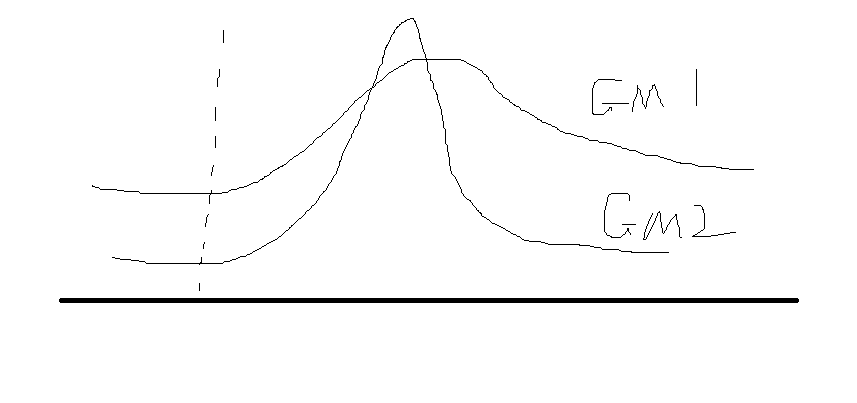最终的分布可能是多个GM根据不同权重叠加的结果。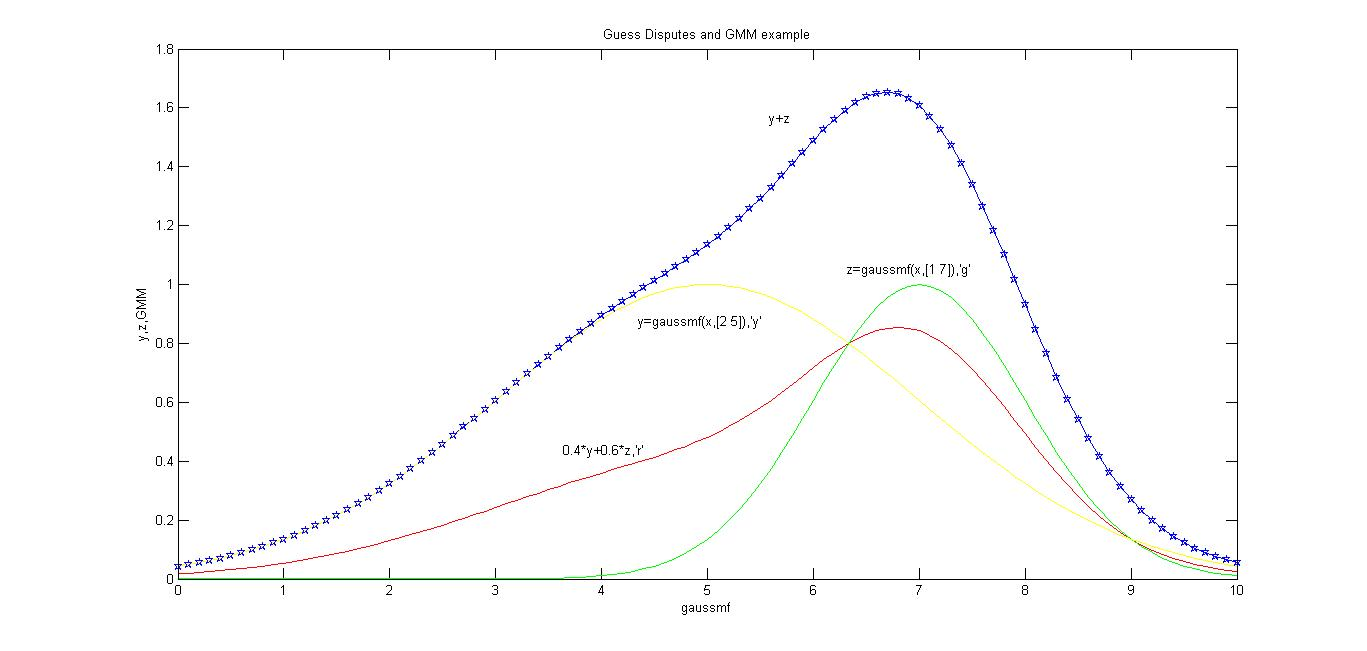好，我们假设有N个数据，利用K个GM来进行描述，每个GM在同一个数据点Xi上所占的比例为πk(k=1,2,3……K)。显然对于一个数据点Xi，有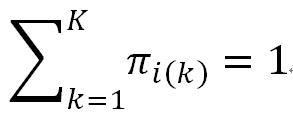我们下面的任务就是确定权重πk（也就确定了GMM中GM的个数，也就是“类”的数目），各类均值Uk和各类协方差σ(k)^2 ，这三个参数。

要解决这样一个问题，想法是这样的：找到一组πkUk和σ(k)^2，让它所确定的概率分布生成这些给定数据点的概率最大。我们可以想象，如果这样一组πkUk，σ(k)^2确定的数据分布与已给数据点完全重合，那么效果最好，也就是我们找到的目标GMM。但是多数情况下，GMM只能达到局部最优，即在局部可以得到收敛解。然而局部最优不一定是全局最优，很有可能得到一个相当差的结果。

典型的例子是人人只为己对整个社会是有害的，会造成双输的局面。我们在这里写博客分享想法就不是出于只为自己考虑的动机~，人只为自己着想，看起来是局部最优，但实际上不是局部最优，长远来看是全局最劣策略。

那既然GMM不是全局最优，为什么还要采用它呢？实际上，即使GMM不是全局最优，通过合理地选定K值和初值Uk，σ(k)^2，也可以得到较好地分类效果。另外，绝对完美的算法很少见，而且改进的GMM也有很多，后面需要用到可以再学习

所以，没必要纠结算法的完美性，而是要关注不完美算法的应用是否能准确刻画或解决问题的某一方面。

下面，回到之前的想法：找到一组πkUk和σ(k)^2，让它所确定的概率分布生成这些给定数据点的概率最大。根据之前的分析，GMM的概率密度等于各类GM在某点出的概率值与其对应权值乘积的结果，即πk*P(x|k)，也就是πk*N(x│u,σ^2 )=1/(σ*√2π) e^((-〖(x-u)〗^2)/(2σ^2 ))，故设在x处GMM的概率密度为P(x)，显然有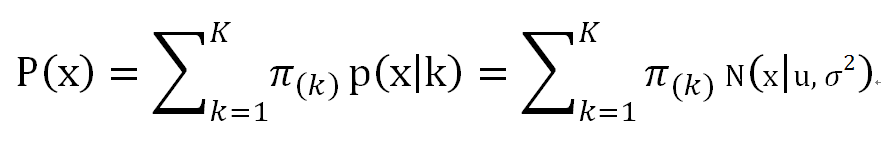式中，πk是第k个GM对数据集的贡献，也就是权重；N(x│u,σ^2 )是在第k个GM时x对应的概率。所以，找到一组πkUk和σ(k)^2，让它所确定的概率分布生成这些给定数据点的概率最大，也就是让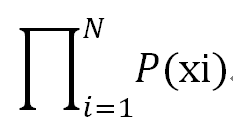最大。

下面的处理显然要按照参数估计里面的极大似然估计。这里有一点要注意的是，通常单个Xi点对应的概率P(Xi)很小，相乘会在计算机里容易造成浮点数下溢的情况，故通常会取对数，即，将乘积写成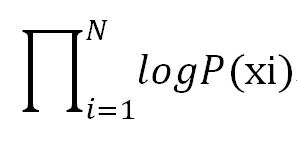下面的问题就是数学问题了，只要求得函数最大值（通常的做法是求导并令导数等于0，然后解方程），亦即找到这样一组参数值。它可以让极大似然函数取得最大值，我们就认为这是合适的参数。因为存在局部最优的情况，如下图。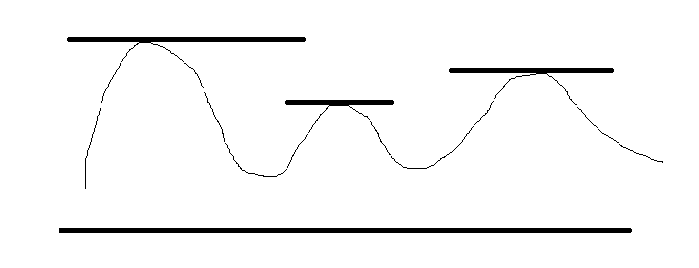故求导为0的点应该不知一个，应该讲满足导数为0的点全部解出来，找到对应的参数，其结果应该是一个导数为0的点对应一个参数组。代入参数组比较，取最大值对应的参数组应该才是正确的。由于GMM在这里的求解多采用EM算法，故很多资料在这里只讲会找出一组参数值，应该是不正确的。既然承认存在局部最优，那应该至少存在2个局部最优的情况（只存在一个局部最优就是全局最优）。

在求解过程中由于对数函数里又有加和，我们没法直接用求导方程的办法直接解方程。多数采用EM（Expection Maximization Algorithm 期望值最大化算法），EM算法类似于K-means求解的步骤。K-means算法的求解就是EM算法的精髓之处。

EM求解分为两步：

第一步假设我们知道K个GM的参数（可以初始化一个，或者基于上一步迭代结果），也就是知道这K个Uk和σ(k)^2,k=1,2,3……K，显然还要确定权值πk。故下面要做的就是估计每个GM的权值。

对于第i个样本，它由第k个GM生成的概率为：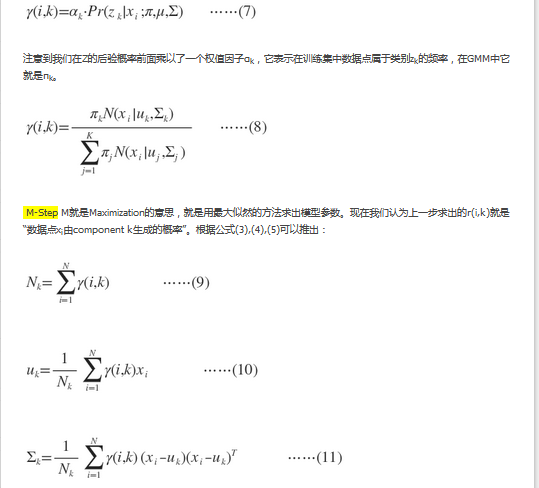公式太繁琐，直接借用其他博主的。

展开全文GMM 算法 聚类
• EM算求解高斯混合模型 1. 如何根据身高判断一个人是男性还是女性 我们首先考虑这么一个问题，假设你拿到了一堆成年人身高的数据，你只知道其中男性的平均身高是要高于女性的，那么你能判断出某个身高的人（比如甲，...

EM算求解高斯混合模型

1. 如何根据身高判断一个人是男性还是女性

我们首先考虑这么一个问题，假设你拿到了一堆成年人身高的数据，你只知道其中男性的平均身高是要高于女性的，那么你能判断出某个身高的人（比如甲，170cm）的人是男性还是女性吗？
或许你已经有些思路了，我可以做一个合理的假设啊，男性和女性的升高都服从正态分布，其中男性升高的正态分布的均值要大于女性，如果我根据给定的数据能做出这两个正太分布的图的话，是不是就能合理的预测身高170的甲的性别了。是的，这种可以抽象为多个正态混合的模型就可以理解为高斯混合模型。

2. 高斯混合模型

如果满足下面条件， X k X_k 表示某一种类型
X 1 ∼ N ( μ 1 , Σ 1 ) X_1 \sim N(\pmb{\mu_1}, \pmb{\Sigma_1})
X 2 ∼ N ( μ 2 , Σ 2 ) X_2 \sim N(\pmb{\mu_2}, \pmb{\Sigma_2})
X 3 ∼ N ( μ 3 , Σ 3 ) X_3 \sim N(\pmb{\mu_3}, \pmb{\Sigma_3})
. . . ...
X K ∼ N ( μ K , Σ K ) X_K \sim N( \pmb{\mu_K}, \pmb{\Sigma_K})
样本X满足
X = { x ( 0 ) , x ( 1 ) , x ( 2 ) , . . . , x ( N − 1 ) } X = \{ \pmb{x^{(0)}}, \pmb{x^{(1)}}, \pmb{x^{(2)}}, ..., \pmb{x^{(N-1)}}\}
x ( j ) ∈ { X 1 , X 2 , X 3 , . . . , X K } x^{(j)} \in \{ X_1, X_2, X_3, ..., X_K\}
就认为样本X是高斯混合模型。
其实简单来说，高斯模型就是描述了这样一个问题。说样本是一堆数据，这些数据都来自K个高斯模型中的一个，但是具体哪个数据来自哪个模型不知道，任意一个高斯模型的参数 μ k , Σ k \pmb{\mu_k}, \pmb{\Sigma_k} 也不知道。如果让我们预测一个新的样本 x \pmb x 最有可能来自哪个模型和（或）这K个模型的参数和（或）在N个样本中，第k个模型贡献了多少个样本，这种问题就可以认为是高斯混合模型的求解。
不过，应该注意的是，上面提到的高斯混合模型并没有说样本 x \pmb x 一定是一维数据，而是任意维度的数据。
下面让我们来回顾上面哪个问题，既然男生的身高我们看成是正态分布，女生的身高也看成是正太分布，那么一群成年人身高构成的样本就构成了一个最简单的高斯混合模型，其中K = 2， 样本x也是一维数据。

3. EM算法初步了解

讲了那么多，终于讲到EM算法了，EM算法（Expectation-Maximization algorithm, EM）翻译成中文是最大期望算法，有过算法学习经验的人应该听到这个名字就大概能对这个算法做一个初步的判断了，它是一种概率模型的算法，它的损失函数应该是最大似然估计函数。EM算法用来解决高斯混合模型的问题，那么如果用EM算法求解高斯混合模型并对未知样本做出分类，那么这种算法是非监督学习算法。一下我们就对EM算法有了初步的了解。

4. 隐藏变量和完全数据的最大似然函数

首先定义隐藏变量，再来解释隐藏变量的含义
γ j k = { 1 , 当 x ( j ) 属 于 第 k 个 模 型 时 0 , e l s e \gamma_{jk} = \begin{cases} 1, &amp; 当x^{(j)}属于第k个模型时 \\ 0, &amp; else \end{cases}
隐藏变量 γ j k \gamma_{jk} 当样本 x i x^{i} 是属于模型k时，则为1，否则为0，理论上来讲， γ j k \gamma_{jk} 的值只能取0或者1，不过由于我们是想要做预测，具体哪个样本来自哪个模型我们很难做100%的断定，因此我们略加引申，以概率的观点来看待 γ j k \gamma_{jk} γ j k \gamma_{jk} 也就表示 x ( j ) x^{(j)} 属于模型k的概率， γ j k \gamma_{jk} 值越大，说明来自模型k的概率越大，当 γ j k \gamma_{jk} 取值为0和1时，表示确定样本 x ( j ) x^{(j)} 来自某个模型k和样本 x ( j ) x^{(j)} 不来自某个模型k
引申后的 γ j k \pmb\gamma_{jk} 的容易得到其性质
0 ≤ γ j k ≤ 1 0 \le \gamma_{jk} \le 1
∑ k = 1 K γ j k = 1 \sum^K_{k = 1}{\gamma_{jk}} = 1
假设样本 x ( j ) x^{(j)} 是一维数据，一阶高斯模型 X k X_k 中的样本x满足的概率密度函数如下("|"后面表示参数，前面表示变量，就是表示给定参数后变量的函数，下同)
f ( x ∣ μ k , σ k 2 ) = 1 2 π σ k ∗ e x p ( − ( x − μ k ) 2 σ k 2 ) f(x|\mu_k, \sigma^2_k) = \frac{1}{\sqrt{2\pi}\sigma_k}*exp(-\frac{(x - \mu_k)^2}{\sigma^2_k})
那么对样本的估计要包含两步， 首先要对样本 x ( j ) x^{(j)} 的完全数据 ( x ( j ) , γ j k ) (x^{(j)},\gamma_{jk}) 做估计得到 p ( x ( j ) , γ j k ) p(x^{(j)},\gamma_{jk}) ,然后按照条件概率公式
p ( x ( j ) ) = ∑ γ j k p ( x ( j ) , γ j k ) p ( γ j k ) p(x^{(j)}) = \sum_{\gamma_{jk}}{p(x^{(j)}, \gamma_{jk})p(\gamma_{jk})}
下面重点研究完全数据的概率密度函数为
p ( x ( j ) , γ j k ∣ α , μ , σ 2 ) = ∏ k = 1 K ( α k ⋅ f ( x ( j ) ∣ μ k , σ k 2 ) ) γ j k p(x^{(j)},\gamma_{jk}|\pmb \alpha, \pmb\mu, \pmb\sigma^2) = \prod_{k = 1}^K{(\alpha_k \cdot f(x^{(j)}|\mu_k, \sigma^2_k))}^{\gamma_{jk}}
其中
α = ( α 1 , α 2 , α 3 , . . . . . . , α K ) \pmb\alpha = (\alpha_1, \alpha_2, \alpha_3, ......, \alpha_K)
μ = ( μ 1 , μ 2 , μ 3 , . . . . . . , μ K ) \pmb\mu = (\mu_1, \mu_2, \mu_3, ......, \mu_K)
σ = ( σ 1 , σ 2 , σ 3 , . . . . . . , σ K ) \pmb\sigma = (\sigma_1, \sigma_2, \sigma_3, ......, \sigma_K)
μ , σ \pmb\mu, \pmb\sigma 这两个变量比较好理解，但是 α \pmb\alpha 是第一次出现，需要解释一下
α k \alpha_k 表示第k个模型中的样本占总样本数量的比例， α \pmb\alpha 代表各模型中的样本占样本总量的比例，概率密度函数表示，容易得到如下的性质
∑ k = 1 K α k = 1 \sum_{k = 1}^K \alpha_k = 1
概率密度函数的解释，其中 α k ⋅ f ( x ( j ) ∣ μ k , σ k 2 ) \alpha_k \cdot f(x^{(j)}|\mu_k, \sigma^2_k) 表示经样本比重调节后的单个样本在全部样本中的修正概率密度，保证了全部来自模型k的样本得到的样本总权重为 α k \alpha_k ( α k ⋅ f ( x ( j ) ∣ μ k , σ k 2 ) ) γ j k (\alpha_k \cdot f(x^{(j)}|\mu_k, \sigma^2_k))^{\gamma_{jk}} 表示单个样本在某个模型中的以概率 γ j k \gamma_{jk} 修正后的概率。
那么全部样本的完全数据的似然估计为
p ( x , γ ∣ α , μ , σ 2 ) = ∏ j = 0 N − 1 ∏ k = 1 K ( α k ⋅ 1 2 π σ k ∗ e x p ( − ( x ( j ) − μ k ) 2 σ k 2 ) ) γ j k p(x, \pmb\gamma|\pmb \alpha, \pmb\mu, \pmb\sigma^2) = \prod_{j = 0}^{N-1}{\prod_{k = 1}^K{(\alpha_k \cdot\frac{1}{\sqrt{2\pi}\sigma_k}*exp(-\frac{(x^{(j)} - \mu_k)^2}{\sigma^2_k}))^{\gamma_{jk}}}}
全部样本的完全数据的对数似然估计为
log ⁡ p ( x , γ ∣ α , μ , σ 2 ) = ∑ k = 1 K ( n k log ⁡ α k + ∑ j = 0 N − 1 γ j k ( log ⁡ ( 1 2 π ) − log ⁡ σ k − 1 2 σ k 2 ( x ( j ) − μ k ) 2 ) ) \log p(x, \pmb\gamma|\pmb \alpha, \pmb\mu, \pmb\sigma^2) = \sum_{k=1}^K{(n_k\log \alpha_k + \sum_{j=0}^{N-1}{\gamma_{jk}(\log(\frac{1}{\sqrt{2\pi}}})-\log \sigma_k - \frac{1}{2\sigma^2_k}(x^{(j)} - \mu_k)^2\big)\Big)}
其中
n k = ∑ j = 0 N − 1 γ j k n_k = \sum_{j=0}^{N-1}{\gamma_{jk}}
γ = [ γ 0 , 1 γ 0 , 2 γ 0 , 3 . . . γ 0 , K γ 1 , 1 γ 1 , 2 γ 1 , 3 . . . γ 1 , K γ 2 , 1 γ 2 , 2 γ 2 , 3 . . . γ 2 , K . . . . . . . . . . . . . . . γ N − 1 , 1 γ N − 1 , 2 γ N − 1 , 3 . . . γ N − 1 , K ] \pmb\gamma = \left[ \begin {matrix} \gamma_{0,1} &amp;\gamma_{0,2} &amp;\gamma_{0, 3} &amp;... &amp;\gamma_{0, K}\\ \gamma_{1,1} &amp;\gamma_{1,2} &amp;\gamma_{1, 3} &amp;... &amp;\gamma_{1, K}\\ \gamma_{2,1} &amp;\gamma_{2,2} &amp;\gamma_{2, 3} &amp;... &amp;\gamma_{2, K}\\ ... &amp;... &amp;... &amp;... &amp;...\\ \gamma_{N-1,1} &amp;\gamma_{N-1,2} &amp;\gamma_{N-1, 3} &amp;... &amp;\gamma_{N-1, K} \end{matrix} \right]

5 迭代公式

根据前面一节的讲解，可以得到高斯混合模型的对数似然函数 log ⁡ p ( x , γ ∣ α , μ , σ 2 ) \log p(x, \pmb\gamma|\pmb \alpha, \pmb\mu, \pmb\sigma^2) ，但是这个函数中多了一个对 γ \pmb\gamma 的估计，按照《统计学习方法》第9章的方法，可以得到极大化样本模型的对数似然估计函数 log ⁡ p ( x ∣ α , μ , σ 2 ) \log p(x|\pmb\alpha, \pmb\mu, \pmb\sigma^2) 等价于极大化 E ( log ⁡ p ( x , γ ∣ α , μ , σ 2 ) ) E(\log p(x, \pmb\gamma|\pmb\alpha, \pmb\mu, \pmb\sigma^2))
E ( log ⁡ p ( x , γ ∣ α , μ , σ 2 ) ) E(\log p(x, \pmb\gamma|\pmb\alpha, \pmb\mu, \pmb\sigma^2)) 式子展开可以得到，
γ ^ j k = α k f ( x ( j ) ∣ μ k , σ k ) ∑ k = 1 K α k f ( x ( j ) ∣ μ k , σ k \hat\gamma_{jk} = \frac{\alpha_kf(x^{(j)}|\mu_k, \sigma_k)}{\sum_{k=1}^K{\alpha_kf(x^{(j)}}|\mu_k, \sigma_k}
并将优化目标对 α , μ , σ 2 \pmb\alpha, \pmb\mu, \pmb\sigma^2 求导最后可以得到
μ ^ k = ∑ j = 0 N − 1 γ ^ j k ⋅ x ( j ) ∑ j = 0 N − 1 γ ^ j k \hat\mu_k = \frac{\sum_{j=0}^{N-1}{\hat\gamma_{jk}}\cdot x^{(j)}}{\sum_{j=0}^{N-1}{\hat\gamma_{jk}}}
σ ^ k 2 = ∑ j = 0 N − 1 γ ^ j k ( x ( j ) − μ k ) 2 ∑ j = 0 N − 1 γ ^ j k \hat\sigma_k^2 = \frac{\sum_{j=0}^{N-1}{\hat\gamma_{jk}(x^{(j)}-\mu_k)^2}}{\sum_{j=0}^{N-1}{\hat\gamma_{jk}}}
α ^ k = ∑ j = 0 N − 1 γ ^ j k N \hat\alpha_k = \frac{\sum_{j=0}^{N-1}{\hat\gamma_{jk}}}{N}
通过不断的迭代 γ ^ j k \hat\gamma_{jk} μ ^ k \hat\mu_k σ ^ k 2 \hat\sigma_k^2 α ^ k \hat\alpha_k ，直到后面三者不再发生明显变动，说明迭代停止，得到预测参数

6 了解更多

上面的过程写的比较简略，很多步骤都有省略，想要详细的推到过程，可以参考李航著《统计学习方法》或访问网站https://blog.csdn.net/jinping_shi/article/details/59613054，这个网站里还对二维高斯混合模型有所讲解，二维混合模型得到的迭代公式和一维情况下的迭代公式基本相同，只是将求方差的方法换成求二维协方差的方法，推广到更高维，算法也基本相同，只是将方差公式推广位多维协方差计算公式
另外，如果想要进一步交流，可以联系邮箱1540838935@qq.com

展开全文em算法 高斯混合模型
•   由于最近要做聚类算法方面的内容，看了很多资料，在高斯混合模型(GMM)这里一直没有一个让我完全推导清楚的、理解的文章。经过三天打鱼两天晒网 不懈努力，总算是有一点自己的理解，我希望尽量通俗地把GMM讲明白...

0. 引言

由于最近要做聚类算法方面的内容，看了很多资料，在高斯混合模型(GMM)这里一直没有一个让我完全推导清楚的、理解的文章。经过三天打鱼两天晒网 不懈努力，总算是有一点自己的理解，我希望尽量通俗地把GMM讲明白，同时也希望尽量详细地对公式进行推导和解释。因此，我会先给出GMM算法的总体步骤，保证拿上先可以直接使用，然后再进行具体的推导和解释。文中可能有一些自己理解不严谨的地方，还请大家指正。

1. 算法初窥

已知样本集是 D = { x 1 , x 2 , . . . , x m } D=\{x_1,x_2,...,x_m\} ，要将这些样本聚成 k k 类。我们认为样本服从混合高斯分布：
p M ( x ) = ∑ i = 1 k α i ⋅ p ( x ∣ μ i , Σ i ) p_M(\bm{x})=\sum_{i=1}^k \alpha_i \cdot p(\bm{x}|\bm{\mu_i}, \bm{\Sigma_i})
其中 p ( x ∣ μ i , Σ i ) = 1 ( 2 π ) n / 2 ∣ Σ i ∣ 1 / 2 e x p { − 1 2 ( x − μ i ) T Σ i − 1 ( x − μ i ) } p(\bm{x}|\bm{\mu_i}, \bm{\Sigma_i})=\frac{1}{_{(2\pi)^{n/2}|\bm{\Sigma_i}|^{1/2}}}exp\{-\frac{1}{2}(\bm{x}-\bm{\mu_i})^T\bm{\Sigma_i}^{-1}(\bm{x}-\bm{\mu_i})\} 是一个多元高斯分布，即一个混合成分；
α i \alpha_i 表示混合系数，即选择第 i i 个混合成分的概率。

第一步 初始化高斯混合分布的模型参数 α i , μ i , Σ i \alpha_i,\bm{\mu_i},\bm{\Sigma_i}
第二步 计算 x j x_j 由各混合成分生成的后验概率，即观测数据 x j x_j 由第 i i 个分模型生成的概率 p ( z j = i ∣ x j ) p(z_j=i|\bm{x_j}) ，并记为 γ j i \gamma_{ji}
γ j i = α i ⋅ p ( x j ∣ μ i , Σ i ) ∑ l = 1 k α l ⋅ p ( x j ∣ μ l , Σ l ) \gamma_{ji}=\frac{\alpha_i\cdot p(\bm{x_j}|\bm{\mu_i},\bm{\Sigma_i})}{_{\sum_{l=1}^{^k}\alpha_l \cdot p(\bm{x_j}|\bm{\mu_l},\bm{\Sigma_l})}}

第三步 计算新的模型参数：

μ i ′ = ∑ j = 1 m γ j i x j ∣ ∑ j = 1 m γ j i ∣ \bm{\mu_i'}=\frac{\sum_{_{j=1}}^m\gamma_{ji}\bm{x_j}^{\color{white}{|}}}{\sum_{_{j=1}}^m\gamma_{ji}^{\color{white}{|}}}

Σ i ′ = ∑ j = 1 m γ j i ( x j − μ i ′ ) ( x j − μ i ′ ) T ∣ ∑ j = 1 m γ j i ∣ \bm{\Sigma_i'}=\frac{\sum_{_{j=1}}^m\gamma_{ji}(\bm{x_j}-\bm{\mu_i'})(\bm{x_j}-\bm{\mu_i'})^{T^{\color{white}{|}}}}{\sum_{_{j=1}}^m\gamma_{ji}^{\color{white}{|}}}

α i ′ = ∑ j = 1 m γ j i ∣ m \alpha_i'=\frac{\sum^{m}_{_{j=1}}\gamma_{ji}^{\color{white}{|}}}{m}

第四步 按照新的模型参数重复2，3步，直到满足停止条件
第五步 将每个样本按照 λ j = arg max ⁡ i ∈ { 1 , 2 , . . . , k } γ j i \lambda_j=\argmax\limits_{i\in\{1,2,...,k\}} \gamma_{ji} 划入对应的簇。即对每个样本来自哪个分模型的概率大就划入哪个分模型的簇中，最终就得到了 k k 个聚类

2. 高斯混合模型的引入

与k-means聚类不同，高斯混合聚类是采用概率模型来刻画聚类结构。实际上我们可以采用任意不同的概率分布模型来进行刻画，高斯分布是最普遍的一种，如下：
高斯分布：
p ( x ) = 1 ( 2 π ) n / 2 ∣ Σ ∣ 1 / 2 e x p [ − 1 2 ( x − μ ) T Σ − 1 ( x − μ ) ] p(\bm{x})=\frac{1}{(2\pi)^{n/2}|\bm{\Sigma}|^{1/2}}exp[-\frac{1}{2}(\bm{x}-\bm{\mu})^T\bm{\Sigma}^{-1}(\bm{x}-\bm{\mu})]
而单高斯分布模型有其局限性，不能完全反映数据分布的特点，因此我们用多个高斯分布的线性叠加来刻画实际样本，其中一个高斯分模型称为一个混合成分。

理论上来说，当叠加的高斯分模型数量足够多时，可以表征任意一种分布。（这其实很好理解，类比足够多微小线段可以逼近任意一条曲线、足够多复指数信号可以描述任意信号…是一样的道理）

高斯混合分布：
p M ( x ) = ∑ i = 1 k α i ⋅ p ( x ∣ μ i , Σ i ) p_M(\bm{x})=\sum_{i=1}^k \alpha_i \cdot p(\bm{x}|\bm{\mu_i}, \bm{\Sigma_i})
我们认为，手里拿到的样本就是根据这个概率分布抽取得到的（或者说“生成的”）
例如，对于第 j j 个样本 x j \bm{x_j} ，就根据 p M ( x j ) = ∑ i = 1 k α j i ⋅ p ( x j ∣ μ i , Σ i ) p_M(\bm{x_j})=\sum_{i=1}^k \alpha_{ji} \cdot p(\bm{x_j}|\bm{\mu_i}, \bm{\Sigma_i}) 得到。

T I P S : \bm{TIPS:}

1. 这里的 p ( x ) p(x) p M ( x ) p_M(x) 指的是概率密度函数，不是概率，在有些概率书上为了区别，用 f ( x ) f(x) 表示，这里都用 p ( x ) p(x) 表示，但心里要清楚其含义。
2. 接上条，所以 p ( x ∣ μ i , Σ i ) p(\bm{x}|\bm{\mu_i},\bm{\Sigma_i}) 不是条件概率，而是概率密度，" ∣ μ i , Σ i |\mu_i,\Sigma_i "只是明确一下这个概率密度函数包含的参变量。实际上它表示的就是上面单高斯分布的 p ( x ) p(x)
3. x \bm{x} 是一条样本，但是有 n n 个维度，因此是一个 n n 维向量。
4. α i > 0 \alpha_i>0 是在生成这条样本时，选择通过第 i i 个分模型来生成的概率，且 ∑ i = 1 k α i = 1 \sum_{i=1}^k\alpha_i=1 。（不能说成"样本来自第 i i 个分模型的概率"，因为这里是一个先验的情况，如果这样说就成了后验了）
5. μ i , Σ i \bm{\mu}_i,\bm{\Sigma}_i 是第 i i 个分模型的参数。其中， μ i \bm{\mu}_i 表示均值，是一个 n n 维向量， Σ i \bm{\Sigma}_i 表示协方差矩阵，是一个 n × n n×n 方阵。

3. 按照高斯混合模型进行聚类划分

上面说了我们认为手里拿到的样本就是通过高斯混合模型抽取得到的，那么反过来我们要怎么把这些样本用高斯混合模型划分成不同的类别呢？
一个很直接的想法自然是按照模型的混合成分划成 k k 类，一个数据最可能从哪个分模型得来就认为属于哪一类。
在这里，我们要引入一个隐变量 z j ∈ { 1 , 2 , . . . , k } z_j\in\{1,2,...,k\} 表示得到样本 x j \bm{x_j} 的高斯分模型。

注：

1. 有的书上用一维向量来表示，即若认为样本 x j \bm{x_j} 来自第2个高斯分模型，则 z j = [ 0 , 1 , 0 , 0 , . . . , 0 ] z_j=[0,1,0,0,...,0] 。 这里直接用数字来表示来自第几个分模型。
2. 根据 z j z_j 的含义很容易看出， P ( z j = i ) P(z_j=i) 表示 x j \bm{x_j} 是通过第 i i 个分模型生成的概率，就是高斯混合模型中的参数 α j i \alpha_{ji}

前面我们说了， α \alpha 是一个先验概念，是从模型到样本的过程。而现在我们在已经拿到了样本的情况下反推其来自哪个分模型，是逆向过程，因此我们用 p M ( z j = i ∣ x j ) p_M(z_j=i|\bm{x_j}) 来表示样本 x j \bm{x_j} 来自第 i i 个分模型的后验概率，并简记为 γ j i \gamma_{ji} 。有：
p M ( z j = i ∣ x j ) = P ( z j = i ) ⋅ p M ( x j ∣ z j = i ) p M ( x j ) = α i ⋅ p ( x j ∣ μ i , Σ i ) ∑ l = 1 k α l ⋅ p ( x j ∣ μ l , Σ l ) \begin{aligned} p_M(z_j=i|\bm{x_j}) & = \frac{P(z_j=i) \cdot p_M(\bm{x_j}|z_j=i)} {p_M(\bm{x_j})} \\ & =\frac{\alpha_i \cdot p(\bm{x_j}|\bm{\mu_i},\bm{\Sigma_i})} {\sum_{l=1}^{k} \alpha_l \cdot p(\bm{x_j}|\bm{\mu_l},\bm{\Sigma_l})} \end{aligned}

注：

1. p M ( x j ∣ z j = i ) p_M(\bm{x_j}|z_j=i) 表示按照第 i i 个高斯分模型生成 x j \bm{x_j} 的概率密度，第 i i 个高斯分模型的参数是 μ i , Σ i \bm{\mu_i},\bm{\Sigma_i} ，故而就等于 p ( x j ∣ μ i , Σ i ) p(\bm{x_j}|\bm{\mu_i},\bm{\Sigma_i})
2. p M ( x j ) p_M(\bm{x_j}) 表示综合所有的混合成分后总的概率密度
3. 上述等式第一行由贝叶斯公式得到
贝叶斯公式： p ( A ∣ B ) = p ( A ) p ( B ∣ A ) p ( B ) p(A|B)=\frac{p(A)p(B|A)}{_{p(B)}}

那么显而易见地，每个样本 x j \bm{x_j} 的簇标记 λ j \lambda_j 如下确定：
λ j = arg max ⁡ i ∈ { 1 , 2 , . . . , k } γ j i \lambda_j=\argmax_{i \in \{1,2,...,k\}}\gamma_{ji}
即， x j \bm{x_j} 来自哪个分模型的概率最大，就认为属于哪一类。

4. 确定高斯混合模型参数

上面已经说了当已知高斯混合模型时，就可以进行聚类的划分，那么如何求解这个模型，得到它的三个参数呢？
我们在这里要用到的是EM算法（期望最大算法）。其实原理很简单：为什么我们能拿到手中的样本，而不是其他数据呢？我们认为这是由于选出这样一组样本的概率最大，所以才运气爆表，被我们拿到手。
由上文知，按照高斯混合模型选出一个样本 x j \bm{x_j} 的概率密度
p M ( x j ) = ∑ i = 1 k α i ⋅ p ( x j ∣ μ i , Σ i ) p_M(\bm{x_j})=\sum_{i=1}^k\alpha_i \cdot p(\bm{x_j}|\bm{\mu_i},\bm{\Sigma_i})
对于手中的 m m 个样本，选到任意一个都是一个独立事件，最终的概率自然是全部相乘，即
∏ j = 1 m p M ( x j ) \prod_{j=1}^mp_M(\bm{x_j})
但是，连乘不好处理，因此一般习惯对它取对数，于是样本集 D D 的最大化对数似然函数就定义如下：
L L ( D ) = ln ⁡ ( ∏ j = 1 m p M ( x j ) ) = ∑ j = 1 m ln ⁡ ( p M ( x j ) ) = ∑ j = 1 m ln ⁡ ( ∑ i = 1 k α i ⋅ p ( x j ∣ μ i , Σ i ) ) \begin{aligned} LL(D) & =\ln(\prod_{j=1}^mp_M(\bm{x_j})) \\ & =\sum_{j=1}^m \ln(p_M(\bm{x_j})) \\ & =\sum_{j=1}^m \ln(\sum_{i=1}^k \alpha_i \cdot p(\bm{x_j}|\bm{\mu_i},\bm{\Sigma_i})) \end{aligned}

只要能求出使 L L ( D ) LL(D) 最大的参数就可以了。
那么怎么求满足要求的参数呢？
我们设参数 θ i = { ( α i , μ i , Σ i ) } \theta_i=\{(\alpha_i,\mu_i,\Sigma_i)\} 能使 L L ( D ) LL(D) 最大化，那么 L L ( D ) LL(D) 对每个参数的偏导数应该为0，但是偏导数为0求出的参数有可能只是局部最优解（ L L ( D ) LL(D) 取极大值或驻点），而不是全局最优解（ L L ( D ) LL(D) 取最大值）。
经过后面的推导，我们可以发现求出的每个参数，都可以用 γ j i \gamma_{ji} 表示。所以，我们在求出了一组模型参数后，按照这种模型得到对应的 γ j i \gamma_{ji} ，再用得到的 γ j i \gamma_{ji} 继续按照偏导数为0的方式求出新的参数。如此循环迭代，直到我们认为足够为止。

至于为什么每次迭代都可以使求得的参数更优，这个问题就不在本文展开叙述了，具体可以参考EM算法的相关资料。

现在我们来具体求解每个参数：

① μ : \bm{\mu}:

∂ L L ( D ) ∂ μ i = 0 ⇒ ∂ ∂ μ i ∑ j = 1 m ln ⁡ ( ∑ i = 1 k α i ⋅ p ( x j ∣ μ i , Σ i ) ) = 0 ⇒ ∑ j = 1 m 1 ∑ l = 1 k α l ⋅ p ( x j ∣ μ l , Σ l ) ⋅ ∂ ∂ μ i [ ∑ l = 1 k α l ⋅ p ( x j ∣ μ l , Σ l ) ] = 0 \begin{aligned} & \frac{\partial LL(D)}{\partial \bm{\mu_i}}=0 \\ & \rArr \frac{\partial}{\partial \bm{\mu_i}}\sum_{j=1}^m \ln (\sum_{i=1}^k \alpha_i \cdot p(\bm{x_j}|\bm{\mu_i},\bm{\Sigma_i}))=0 \\ & \rArr \sum_{j=1}^m\frac{1}{\sum_{l=1}^k \alpha_l \cdot p(\bm{x_j}|\bm{\mu_l},\bm{\Sigma_l})} \cdot \frac{\partial}{\partial \bm{\mu_i}}[\sum_{l=1}^k\alpha_l \cdot p(\bm{x_j}|\bm{\mu_l},\bm{\Sigma_l})]=0 \end{aligned}

(这里因为对 μ i \bm{\mu_i} 求偏导，为了避免混淆，将求和变量写成 l l )
对 ∂ ∂ μ i [ ∑ l = 1 k α l ⋅ p ( x j ∣ μ l , Σ l ) ] \frac{\partial}{\partial \bm{\mu_i}}[\sum_{l=1}^k\alpha_l \cdot p(\bm{x_j}|\bm{\mu_l},\bm{\Sigma_l})] 来说，只有当 l = i l=i 时，包含 μ i \mu_i 的内容，其余对 μ i \mu_i 求偏导均为0，可以舍去，则继续推导如下：

⇒ ∑ j = 1 m 1 ∑ l = 1 k α l ⋅ p ( x j ∣ μ l , Σ l ) ⋅ ∂ ∂ μ i [ α i ⋅ p ( x j ∣ μ i , Σ i ) ] = 0 \rArr \sum_{j=1}^m\frac{1}{\sum_{l=1}^k \alpha_l \cdot p(\bm{x_j}|\bm{\mu_l},\bm{\Sigma_l})} \cdot \frac{\partial}{\partial \bm{\mu_i}}[ \alpha_i \cdot p(\bm{x_j}|\bm{\mu_i},\bm{\Sigma_i})]=0

其中，

∂ ∂ μ i [ α i ⋅ p ( x j ∣ μ i , Σ i ) ] = ∂ ∂ μ i { α i 1 ( 2 π ) n / 2 ∣ Σ i ∣ 1 / 2 exp ⁡ [ − 1 2 ( x j − μ i ) T Σ i − 1 ( x j − μ i ) ] } = α i exp ⁡ [ − 1 2 ( x j − μ i ) T Σ i − 1 ( x j − μ i ) ] ( 2 π ) n / 2 ∣ Σ i ∣ 1 / 2 ∂ ∂ μ i [ − 1 2 ( x j − μ i ) T Σ i − 1 ( x j − μ i ) ] = α i ⋅ p ( x j ∣ μ i , Σ i ) ⋅ ( x j − μ i ) \begin{aligned} & \frac{\partial}{\partial \bm{\mu_i}}[ \alpha_i \cdot p(\bm{x_j}|\bm{\mu_i},\bm{\Sigma_i})] \\ & =\frac{\partial}{\partial \bm{\mu_i}}\{\alpha_i\frac{1}{(2\pi)^{n/2}|\bm{\Sigma_i}|^{1/2}} \exp[-\frac{1}{2}(\bm{x_j}-\bm{\mu_i})^T\bm{\Sigma_i}^{-1}(\bm{x_j}-\bm{\mu_i})]\} \\ & =\alpha_i\frac{\exp[-\frac{1}{2}(\bm{x_j}-\bm{\mu_i})^T\bm{\Sigma_i}^{-1}(\bm{x_j}-\bm{\mu_i})]}{(2\pi)^{n/2}|\bm{\Sigma_i}|^{1/2}} \frac{\partial}{\partial\bm{\mu_i}}[-\frac{1}{2}(\bm{x_j}-\bm{\mu_i})^T\bm{\Sigma_i}^{-1}(\bm{x_j}-\bm{\mu_i})] \\ & =\alpha_i\cdot p(\bm{x_j}|\bm{\mu_i},\bm{\Sigma_i})\cdot(\bm{x_j}-\bm{\mu_i}) \end{aligned}

这里是向量/矩阵对另一个向量求导，不是标量求导，具体可以参考矩阵求导相关资料

因此继续推导如下：
⇒ ∑ j = 1 m α i ⋅ p ( x j ∣ μ i , Σ i ) ∑ l = 1 k α l ⋅ p ( x j ∣ μ l , Σ l ) ( x j − μ i ) = 0 ⇒ ∑ j = 1 m p M ( z j = i ∣ x j ) ( x j − μ i ) = 0 ⇒ ∑ j = 1 m γ j i ⋅ ( x j − μ i ) = 0 ⇒ ∑ j = 1 m γ j i x j = ∑ j = 1 m γ j i μ i ⇒ μ i = ∑ j = 1 m γ j i x j ∑ j = 1 m γ j i \begin{aligned} & \rArr \sum_{j=1}^m\frac{\alpha_i \cdot p(\bm{x_j}|\bm{\mu_i},\bm{\Sigma_i})}{\sum_{l=1}^k \alpha_l \cdot p(\bm{x_j}|\bm{\mu_l},\bm{\Sigma_l})} (\bm{x_j}-\bm{\mu_i})=0 \\ & \rArr \sum_{j=1}^m p_M(z_j=i|\bm{x_j})(\bm{x_j}-\bm{\mu_i})=0 \\ & \rArr \sum_{j=1}^m \gamma_{ji}\cdot (\bm{x_j}-\bm{\mu_i})=0 \\ & \rArr \sum_{j=1}^m \gamma_{ji}\bm{x_j}=\sum_{j=1}^m\gamma_{ji}\bm{\mu_i} \\ & \rArr \bm{\mu_i}=\frac{\sum_{j=1}^m \gamma_{ji}\bm{x_j}}{\sum_{j=1}^m\gamma_{ji}} \end{aligned}

至此，参数 μ i \bm{\mu_i} 迭代公式得到。

② Σ : \bm{\Sigma}:

同理，由
∂ L L ( D ) ∂ Σ i = 0 \frac{\partial LL(D)}{\partial \bm{\Sigma_i}}=0
推得：
Σ i = ∑ j = 1 m γ j i ( x j − μ i ) ( x j − μ i ) T ∑ j = 1 m γ j i \bm{\Sigma_i}=\frac{\sum_{j=1}^m\gamma_{ji}(\bm{x_j}-\bm{\mu_i})(\bm{x_j}-\bm{\mu_i})^T}{\sum_{j=1}^m\gamma_{ji}}

③ α : \alpha:

求 α \alpha 的过程略有不同，因为除了要使 L L ( D ) LL(D) 最大化以外， α \alpha 还要满足它自身的条件： α i ≥ 0 , ∑ i = 1 k α i = 1 \alpha_i ≥0,\sum_{i=1}^k\alpha_i=1
这是一个有条件的极值问题，我们要用拉格朗日乘数法来求解（具体可以参考拉格朗日乘数法求极值的相关资料）
相当于将 L L ( D ) LL(D) 求极值问题转化为 L L ( D ) + λ ( ∑ i = 1 k α i − 1 ) LL(D)+\lambda(\sum_{i=1}^k\alpha_i -1) 求极值的问题，然后依然对 α i \alpha_i 求导为0，由此得到：
α i = 1 m ∑ j = 1 m γ j i \alpha_i=\frac{1}{m}\sum_{j=1}^m\gamma_{ji}

至此，高斯混合模型聚类的所有参数公式均已得到，之后只要不断迭代，并按照文章第3节中的划分方式来进行聚类划分即可。

最后，可以再回头看看文章第1节的算法总结。

参考资料

1. 周志华，机器学习，清华大学出版社，2016
2. 李航，统计学习方法，清华大学出版社，2012

展开全文机器学习 聚类 算法 人工智能
• 高斯混合模型（GMM）和EM算法详解

千次阅读 多人点赞 2020-03-09 02:27:49
1. 单高斯模型GSM（一维） 单高斯模型很简单，大家也很清楚，这里不做过多的解释，如不明白可自行百度。如图 概率密度函数为： 2.单高斯模型（多维，以二维为例） 二维高斯分布图像如下 ...人工智能 机器学习 算法
• K-means算法EM步骤如下：给定K的值，代表有K个不同的类别。对每一个类别，猜测其中心点。 在已知K个中心点的情况下，计算每个点到这K的中心点的距离，距离最小的那个中心点所代表的类就是该点所属的类别，这样对所有...
• 一个利用em算法，求解高斯混合模型的聚类源程序！
• 最重要的背景识别算法之一是高斯混合模型算法（GMM）。在高斯混合模型的实现上会导致整个系统的处理能力降低。 可训练分割适用于提高处理能力。 在对性能进行分析和评估后，我们总结出几个有希望的未来研究方向。
• 本文详细介绍了EM算法步骤 分析，以及应用与高斯混合模型和隐马尔可夫过程参数估计的详细过程，英文版
• 最近在看晓川老（shi）师(shu)的博士论文，接触了混合高斯模型（Gaussian mixture model, GMM）和EM(Expectation Maximization)算法，不禁被论文中庞大的数学公式所吓退。本文通过查阅相关资料，在复杂巧妙的推理...EM算法 极大似然估计
• 高斯混合模型(Gaussian Mixture Model)简称GMM, 高斯混合模型使用K个高斯分布的结合组成的概率分布模型，并使用了期望最大(Expectation Maximization，简称EM)算法进行训练。其理论基础是：K个高斯分布的结合组成的...机器学习 混合高斯模型 聚类算法
• 文章目录一、总结K均值算法步骤二、...十、高斯混合模型与K均值算法比较十一、如何快速收敛数据量大的k-means算法？ 一、总结K均值算法步骤 二、如何合理选择K值？ 手肘法 我们可以尝试不同的K值，并将不同K值所对K均值算法 EM算法 高斯混合模型 百面机器学习
• EM算法与高斯混合模型

千次阅读 2018-08-24 10:14:14
EM算法高斯混合模型 Jensen不等式 极大似然估计 EM算法 问题描述 EM算法基本步骤 EM算法推导 高斯混合模型 高斯分布 EM算法求解GMM的步骤 EM算法高斯混合模型 EM算法（The Expectation-Maximization ...机器学习
• 1.介绍 实验通过python用两个不同的高斯分布模型产生随机数据，然后采用EM算法对数据进行迭代将数据分成两类，得到两个高斯模型分布。 2.高斯混合模型 ...高斯混合模型的概率分布可以表示如下...高斯混合模型
• 看了西关书的聚类算法算法原理很容易明白，接下来就是整理成自己的理解思路，然后一步一步来实现算法，那么就来做吧。 Guassian代码下载链接 ok啦，接下来不废话上代码（Matlab发布形式） %Fucntion :...算法
• 上机器学习课学到EM算法，通过查阅西瓜书等资料理解了算法的推导过程于是形成了总结，在此进行分享。针对机器学习初学者，内容主要是：EM算法以及GMMs模型算法求解步骤的推导证明
• 高斯混合模型(GMM)和EM算法

万次阅读 多人点赞 2018-07-16 15:54:04
高斯混合模型使用了期望最大(Expectation Maximization， 简称EM)算法进行训练，故此我们在了解GMM之后，也需要了解如何通过EM算法训练(求解)GMM。 二、高斯混合模型(GMM)    在了解高斯混合模型之前，...
• I . 高斯混合模型 ( 样本 -> 模型 ) II . 高斯混合模型 ( 模型 -> 样本 ) III . 高斯混合模型 与 K-Means 迭代过程对比 IV . 高斯混合模型 聚类分析 步骤 ( 1 ) ... 高斯混合模型 聚类分析 步骤 ( 3 ) 更新参数 平均值高斯混合模型 K-Means 聚类 数据挖掘
• 所以在聚类时，我们希望将数据划分为一些簇时，可以假设不同簇中的样本各自服从不同的高斯分布，由此得到的聚类算法称为高斯混合模型高斯混合模型 假设数据可以看作多个高斯分布中生成出来的，每个分模型有自己...高斯混合模型 EM算法 聚类 K-means 机器学习
• 这正是高斯混合模型（或简称为GMM）试图做的。现在让我们进一步讨论这个方法。 定义 高斯混合函数是由几个高斯函数组成的函数，每个由k∈{1，…，k}标识，其中k是我们数据集的聚类数。每个高斯函数由以下参数...
• 高斯混合模型(GMM)是一种常用的聚类模型，通常我们利用最大期望算法(EM)对高斯混合模型中的参数进行估计。 1. 高斯混合模型(Gaussian Mixture models, GMM) 高斯混合模型（Gaussian Mixture Model，GMM）是一种...
• 高斯混合模型意味着每个数据点（随机）从 C 类数据之一中抽取，概率 p_i 从第 i 类中抽取，并且每个类都分布为具有平均标准差 mu_i 和 sigma_i 的高斯分布。 给定从这种分布中提取的一组数据，我们试图估计这些未知...matlab
• 为解决复杂场景中的前景目标提取问题，提出一种应用于复杂变化场景中的基于混合高斯模型的自适应前景提取方法。该方法可以对视频帧中每个像素的高斯分布数进行动态控制，并且通过在线期望最大化( EM) 算法高斯...
• EM算法(以高斯混合模型GMM为例) 假 设 我 们 现 在 有 一 堆 身 高 数 据 ， 而 且 假 定 身 高 和 人 类 种 族 无 关 ， 要 求 估 计 出 一 个 身 高 模 型 ? 如 何 估 计 ？ {\color{red}假设我们现在有一堆身高...极大似然估计 EM算法 高斯混合模型
• 图像处理之高斯混合模型

万次阅读 2017-05-26 17:15:17
图像处理之高斯混合模型 一：概述高斯混合模型(GMM)在图像分割、对象识别、视频分析等方面均有应用，对于任意给定的数据样本集合，根据其分布概率， 可以计算每个样本数据向量的概率分布，从而根据概率分布对其进行...高斯混合模型 GMM 图像处理 机器学习
• 什么是高斯分布？ 高斯分布也叫正态分布，也就是常态分布，什么意思呢？比如说男性的身高，假如说有10000个男性的身高，如果再坐标系上标记出来就是一个正态分布，如果形状还不是和上面的图形一样，那说明数据量还...
• EM算法（expectation maximization algorithm）分为E步和M步，其中E-step主要通过观察数据和现有模型来估计参数，然后用这个估计的参数值来计算似然函数的期望值；而 M-Step 是寻找似然函数最大化时对应的参数。由于...机器学习 算法 可视化
• 使用 混合高斯模型 对视频背景进行建模，实现视频的背景前景分离； 使用 EM 算法迭代估计混合高斯模型参数； 使用Matlab 实现整个视频动静分离过程。matlab 混合高斯模型 EM算法 计算机视觉
• 高斯混合模型/前景分割算法

千次阅读 2017-11-10 09:57:52ubuntu python opencv 算法 高斯混合模型...